Courses

# Test: Shear Force & Bending Moment - 2

## 10 Questions MCQ Test Solid Mechanics | Test: Shear Force & Bending Moment - 2

Description
This mock test of Test: Shear Force & Bending Moment - 2 for Civil Engineering (CE) helps you for every Civil Engineering (CE) entrance exam. This contains 10 Multiple Choice Questions for Civil Engineering (CE) Test: Shear Force & Bending Moment - 2 (mcq) to study with solutions a complete question bank. The solved questions answers in this Test: Shear Force & Bending Moment - 2 quiz give you a good mix of easy questions and tough questions. Civil Engineering (CE) students definitely take this Test: Shear Force & Bending Moment - 2 exercise for a better result in the exam. You can find other Test: Shear Force & Bending Moment - 2 extra questions, long questions & short questions for Civil Engineering (CE) on EduRev as well by searching above.
QUESTION: 1

Solution:
QUESTION: 2

### The beam is loaded as shown in figure. Select the correct BM diagram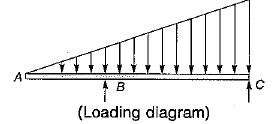Solution:

BMD will be of 2nd degree, so the correct answer is (d).

QUESTION: 3

### The structure shown in the figure below will be stable if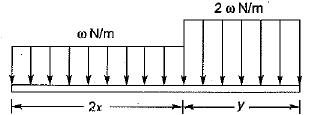Solution: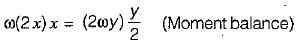∴  2x2 = y2

QUESTION: 4

A beam 8 m long, simply supported at the ends, carries a point load of 1000 N at the mid-span. The bending moment under the load is

Solution: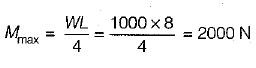QUESTION: 5

A simply supported beam is loaded as shown in the above figure below.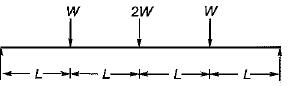The maximum shear force in the beam will be

Solution: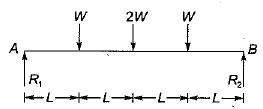Taking moment about A = 0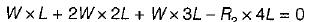R2 = 2W
But, ∑Fv = 0
⇒ W + 2W + W - R1 - R2 = 0
⇒ R1 = 4W - 2W = 2W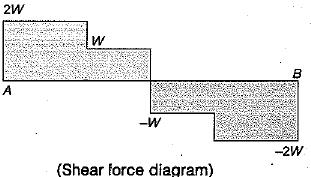QUESTION: 6

Which one of the following portions of the loaded beam shown in the given figure is subjected to pure bending?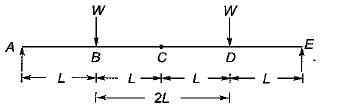Solution:

Since the given beam is loaded symmetrically, therefore the reactions at each suportwill be equal i.e. W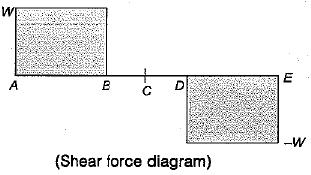In section BD, shear force is zero. Hence this section subjected to pure bending.

QUESTION: 7

The point of contraflexure in a beam is at the location where the

Solution:
QUESTION: 8

The rate of change of bending moment is equal to

Solution:

M = V · x
dM/dx = V
i.e. Rate of change of bending moment is shear force.

QUESTION: 9

The BM diagram of the beam shown in figure is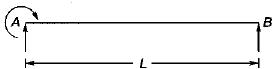Solution:

Taking moments about A. We get
M - R2 + L = 0
⇒ R2 = M/L
Since these is no vertical loading hence the reactions are equal and oposite.
Bending moment is given by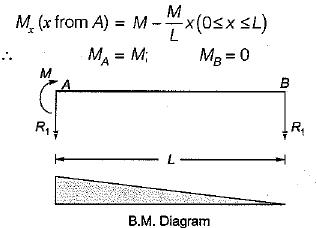QUESTION: 10

A cantilever carrying uniformly distributed load W over its full length is propped at its free end such that it is at the level of the fixed end. The bending moment will be zero at its free end and also at

Solution: